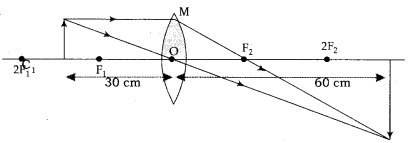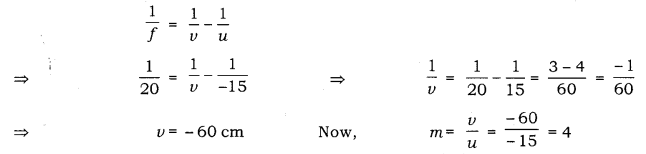# The image of a candle flame placed at a distance of 30 cm from a spherical lens is formed

The image of a candle flame placed at a distance of 30 cm from a spherical lens is formed on a screen placed on the other side of the lens at a distance of 60 cm from the optical centre of the lens. Identify the type of lens and calculate its focal length. If the height of the fl^rne is 3 cm, find the height of its image.Since the image is formed on the screen, the image is real. A concave lens cannot form a real image. Therefore, the lens is convex.
Focal length of the convex lens, f = ?
Object distance, u = - 30 cm
Image distance, V = + 60 cm